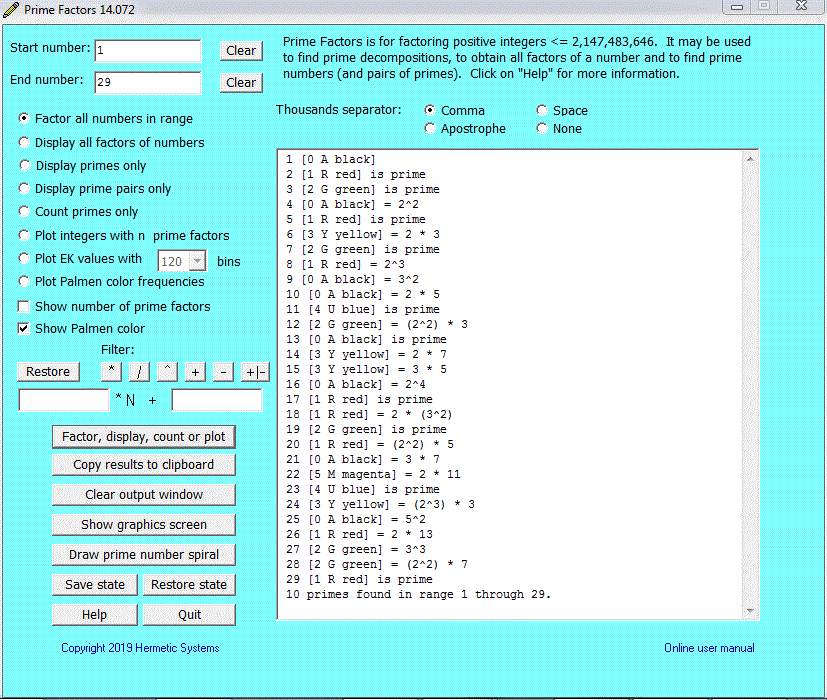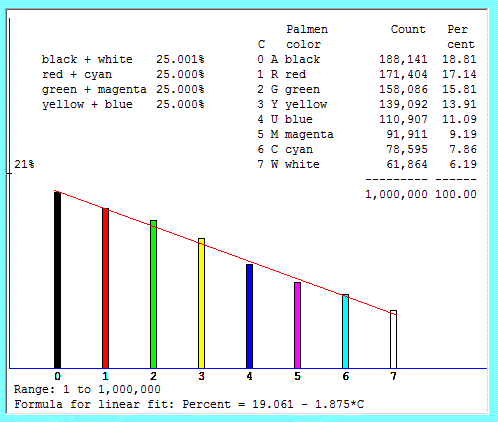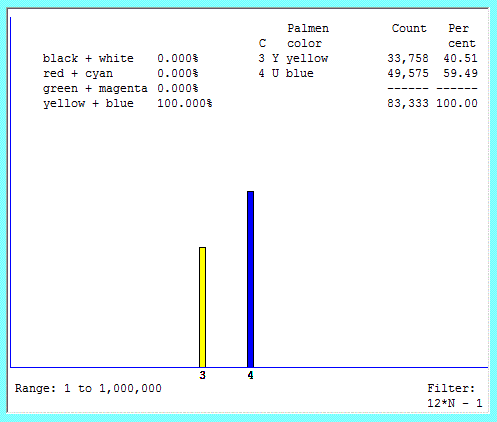Prime Factors Plotting the Palmen Color Frequencies

Prime Factors also computes the Palmen color of a number. There are eight Palmen colors: black, red, green, yellow, blue, magenta, cyan and white. Each natural number has a unique color, and the color of a product of two numbers is determined by the colors of the two numbers (and the Palmen colors form a group). For a complete explanation see Karl Palmen's Colours of Numbers.

The following are samples of the output obtained when the Show Palmen color box is checked:A prime pair consists of either a blue number and a black number or a red number and a green number, as illustrated below.Some Palmen colors occur more frequently than others. Black is the most common and white is the least common. What of the other six Palmen colors? The Prime Factors software allows us to explore their relative frequencies. When we plot the first million positive integers we obtain:It is possible to use a filter when plotting Palmen color frequencies. This allows the experimental discovery of propositions about Palmen colors which are likely to be true although need to be proved mathematically. For example, with the filter 12*N - 1 and the range of integers from 1 through 10,000,000 we obtain:which, of course, strongly suggests that all positive integers of the form 12*N - 1 are either yellow or blue.

The proportion of yellow numbers increases very slowly (41.63% by 20,000,000, 41.97% by 63,000,000). The proportion seems to be given by the expression 36.785*N0.0074, implying that it reaches 50% for N = 1018. Of course, this expression cannot be true for indefinitely large N, since in that case the proportion would exceed 100% for N > 1059. Clearly there is an upper bound (and so an exact limit) to the proportion of yellow numbers (of the form 12*N - 1) as N approaches infinity. Is it 50% exactly? Or does it perhaps exceed 50%?